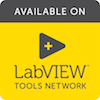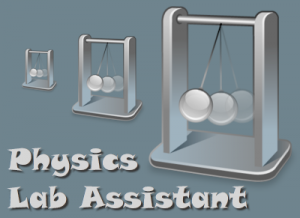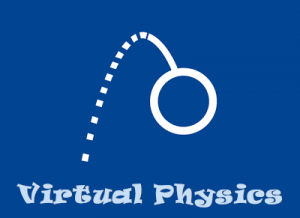### LabVIEW ApplicationsThe following LabVIEW applications have been developed by Doug Harper for use in teaching physics.  They are published on National Instruments’ LabVIEW Tools Network for anyone to use for free.

Click a program icon for additional information on each application.

• ### Physics Lab Assistant

Physics Lab Assistant is an easy to use, versatile, and computationally powerful data acquisition and analysis application that works with National Instruments hardware including the myDAQ, NI ELVIS, or any DAQmx-based device.

Physics Lab Assistant was designed for use in the WKU University Physics Laboratory to support new learning objectives for our curriculum, specifically that students would be able to measure physical quantities using sophisticated data acquisition and be able to develop their own experimental procedures. We wanted the application to be easy to use, versatile, computationally powerful, and to support a scaffolded approach as new concepts were introduced. The application, which was developed using LabVIEW, has proven to be very successful in our laboratory and has recently been made available to a wider audience through the LabVIEW Tools Network.Physics Lab Assistant is flexible enough to be used for any of our experiments and scalable to work with simple to complex experiments. It allows the students to design their experiment by building a series of waveforms (data evenly spaced in time) from sensor measurements and post-acquisition calculations. Students define acquired waveforms that are measured directly from sensors (such as position, force, temperature), derived waveforms that are derived from these using derivatives, integrals, or Fourier transforms (such as velocity, acceleration, impulse), and calculated waveforms that are built from any of the previous using simple algebra (such as momentum, kinetic energy, potential energy, total energy). Finally students can compute scalar quantities such as average, slope, standard deviation, etc. from a selected region of any of the waveform data. Once an experiment is set up in the software it is easy for the students to perform repeated trials to test different experimental parameters.

• ### Virtual Physics

Virtual Physics is tool for numerically integrating the differential equations of motion for many different situations that arise in physics. It is useful both when a solution can and cannot be obtained by standard analytical techniques.

Virtual Physics is tool for numerically integrating the differential equations of motion for many different situations that arise in physics. It can be useful for introductory students who have not yet taken differential equations by allowing them to investigate important problems in physics that they are not mathematically prepared to solve.   It also allows advanced students to study problems for which analytical solutions do not exist.The equations of motion for a classical system obtained either from Newton’s Second Law or the Euler-Lagrange equation are generally second-order differential equations. By introducing intermediate variables these equations can be written as a system of first-order differential equations and then entered symbolically into Virtual Physics. Values for any constants can be specified and Virtual Physics will solve for the unknown coordinates and velocities in the equations of motion using Euler, Runge-Kutta, or Cash-Karp algorithms. Additional waveform quantities to be calculated following the integration, such as potential or kinetic energies, can be defined from the coordinates, velocities, and constants. All of the integrated and calculated waveforms can be displayed graphically or in tabular form and can be exported to tab-delimited or comma-separated-value files.

• ### Digital Oscilloscope

Coming Soon ...